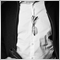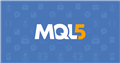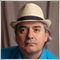# Define Margin per open Position29

Hello everyone.

I really hope to get some hints where to dig further. Till now i could not mange to find a way to calculate the Margin of open Positions while looping through them. The only thing i found was Lot-Size regarding Risk-Management. But was not able to build a connection from that to the Margin.

Thank a lot in advance for any hint with further information on what to look at.

Best regards.29

So i hit two different Solutions:

```if(OrderCalcMargin(ORDER_TYPE_BUY,_Symbol,0.01,SymbolInfoDouble(_Symbol,SYMBOL_ASK),u)
&& OrderCalcMargin(ORDER_TYPE_SELL,_Symbol,0.01,SymbolInfoDouble(_Symbol,SYMBOL_BID),d))```

and

MarginCheck from CAccountInfo

But those calculated values differ from the Margin Shown in the Trade-Toolbox. Maybe someone has a bit of time to explain me why.1538

Pr02type:

So i hit two different Solutions:

and

MarginCheck from CAccountInfo

But those calculated values differ from the Margin Shown in the Trade-Toolbox. Maybe someone has a bit of time to explain me why.

May I try to answer by posting some code....

```        /*
*********************************************************************************************************
*  Margin calculator                                                                                                                                                                    *
*********************************************************************************************************
*/

double mm_calc_margin_required(const double _vol = NULL, const bool bShort = false)
{
// Local init

const string    account_currency        = AccountInfoString(ACCOUNT_CURRENCY);
const string    margin_currency         = SymbolInfoString(Symbol(), SYMBOL_CURRENCY_MARGIN);
const string    profit_currency         = SymbolInfoString(Symbol(), SYMBOL_CURRENCY_PROFIT);
const double    vol_size                = SymbolInfoDouble(Symbol(), SYMBOL_TRADE_CONTRACT_SIZE) * MathRange(_vol, SymbolInfoDouble(Symbol(), SYMBOL_VOLUME_MIN), SymbolInfoDouble(Symbol(), SYMBOL_VOLUME_MAX));
bool            rev_quote       	= (profit_currency == account_currency);
double          calc_price      	= NULL;
string          calc_currency   	= NULL;

MqlTick tick = {0};
ZeroMemory(tick);

// Profit currency equals account currency

if(profit_currency == account_currency)
{ calc_currency = Symbol(); }

// Margin currency equals account currency

if(margin_currency == account_currency)
{
// Calculation currency
calc_currency = Symbol();

// Just return the contract value, multiplied by the number of lots
return(mm_calc_margin_mode(vol_size));
}

// Cross currency pair
// Find calculation currency

if( (StringLen(calc_currency) < 2)
&& (!mm_calc_margin_currency(margin_currency, account_currency, calc_currency, rev_quote)))
{ return(NULL); }

// Quote of last calculation currency

SymbolInfoTick(calc_currency, tick);

// Evaluate price value

calc_price      = (tick.ask * (bShort && rev_quote))
+ ((1.0 / tick.bid) * (bShort && !rev_quote))
+ (tick.bid * (!bShort && rev_quote))
+ ((1.0 / tick.ask) * (!bShort && !rev_quote));

// Calculate required margin
// Return
return(mm_calc_margin_mode(vol_size, bShort) * calc_price);
}

const double mm_calc_margin_mode(const double vol_size, const bool bShort = false)
{
// Local init

const int               account_leverage        = (int)AccountInfoInteger(ACCOUNT_LEVERAGE);
const double            _price                  = (bShort) ? SymbolInfoDouble(Symbol(), SYMBOL_BID) : SymbolInfoDouble(Symbol(), SYMBOL_ASK);
double                  margin_rate             = NULL;
double                  maintenance_rate        = NULL;

if(!SymbolInfoMarginRate(Symbol(), ((bShort) ? ORDER_TYPE_SELL : ORDER_TYPE_BUY), margin_rate, maintenance_rate))
{ return(NULL); }

// Select calculation type

{
case SYMBOL_CALC_MODE_FOREX:                                    return((vol_size / account_leverage) * margin_rate);
case SYMBOL_CALC_MODE_FOREX_NO_LEVERAGE:                        return(vol_size * margin_rate);
case SYMBOL_CALC_MODE_CFD:                                      return(vol_size * _price * margin_rate);
case SYMBOL_CALC_MODE_CFDINDEX:                                 return((vol_size * _price) * (SymbolInfoDouble(Symbol(), SYMBOL_POINT) / SymbolInfoDouble(Symbol(), SYMBOL_TRADE_TICK_SIZE)) * margin_rate);
case SYMBOL_CALC_MODE_CFDLEVERAGE:                              return((vol_size * _price) / (account_leverage * margin_rate));
case SYMBOL_CALC_MODE_EXCH_STOCKS:                              return(vol_size * _price * margin_rate);

// Not implemented
case SYMBOL_CALC_MODE_FUTURES:
case SYMBOL_CALC_MODE_EXCH_FUTURES:
case SYMBOL_CALC_MODE_EXCH_FUTURES_FORTS:
case SYMBOL_CALC_MODE_EXCH_BONDS:
case SYMBOL_CALC_MODE_EXCH_STOCKS_MOEX:
case SYMBOL_CALC_MODE_EXCH_BONDS_MOEX:
case SYMBOL_CALC_MODE_SERV_COLLATERAL:
break;
}

// Return error
return(NULL);
}

const bool mm_calc_margin_currency(const string margin_currency, const string profit_currency, string& calculation_currency, bool& rev_quote)
{
// Local init

bool    custom_symbol           = false;
string  symbolname              = NULL;
string  m_cur                   = NULL;
string  p_cur                   = NULL;

// Initially try guess a symbol

if( (SymbolExist(margin_currency + profit_currency, custom_symbol))
&& (!custom_symbol))
{ calculation_currency = margin_currency + profit_currency; rev_quote = true; return(true); }

if( (SymbolExist(profit_currency + margin_currency, custom_symbol))
&& (!custom_symbol))
{ calculation_currency = profit_currency + margin_currency; rev_quote = false; return(true); }

// Search all available symbols for a match

for(int cnt = 0; (cnt < SymbolsTotal(false)) && !IsStopped(); cnt++)
{
// Get symbol name
symbolname = SymbolName(cnt, false);

// Get margin currency
m_cur = SymbolInfoString(symbolname, SYMBOL_CURRENCY_MARGIN);

// Get profit currency (profit on price change)
p_cur = SymbolInfoString(symbolname, SYMBOL_CURRENCY_PROFIT);

// Check if found
if(      ( (m_cur == margin_currency)
&& (p_cur == profit_currency))
||      ( (p_cur == margin_currency)
&& (m_cur == profit_currency)) )
{
calculation_currency = symbolname;
if(SymbolExist(calculation_currency, custom_symbol) && (!custom_symbol))
{
rev_quote = (m_cur == margin_currency);
return(true);
}
}
}

// Return failure
return(false);
}

double MathRange(const double value, const double min, const double max)
{ return((value * ((value >= min) && (value <= max))) + (min * (value < min)) + (max * (value > max))); }
```29

Dominik Egert:

May I try to answer by posting some code....

Hello Dominik,

thank you very much for that explanation with a code. I like that way a lot more, cause if gives me a direct example. I might need a bit to understand that whole function. It is working really good.

I appreciate your help. And the opportunity to learn something new by a direct example. Hoping that this will be helpful for others, too.

Kind regards.1538

Welcome1538

Pr02type:

Hello Dominik,

thank you very much for that explanation with a code. I like that way a lot more, cause if gives me a direct example. I might need a bit to understand that whole function. It is working really good.

I appreciate your help. And the opportunity to learn something new by a direct example. Hoping that this will be helpful for others, too.

Kind regards.

If you are eager, you could try to implement the missing calculation formulas from this site:

Id be happy if you would share them with me, I have not had the ambition to finish them jet....

https://www.mql5.com/de/docs/constants/environment_state/marketinfoconstants#enum_symbol_calc_modeDokumentation zu MQL5: Konstanten, Enumerationen und Strukturen / Medium Zustand / Information über das Symbol
• www.mql5.com
Information über das Symbol - Medium Zustand - Konstanten, Enumerationen und Strukturen - Nachschlagewerk MQL5 - Nachschlagewerk über die Sprache des algothitmischen/automatischen Handels für MetaTrader 55730

Dominik Egert: If you are eager, you could try to implement the missing calculation formulas from this site: Id be happy if you would share them with me, I have not had the ambition to finish them jet....

https://www.mql5.com/de/docs/constants/environment_state/marketinfoconstants#enum_symbol_calc_mode

Here is the link for the English version for other users that don't understand German ...Documentation on MQL5: Constants, Enumerations and Structures / Environment State / Symbol Properties
• www.mql5.com
Symbol Properties - Environment State - Constants, Enumerations and Structures - MQL5 Reference - Reference on algorithmic/automated trading language for MetaTrader 5